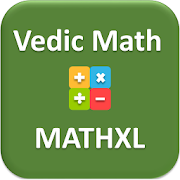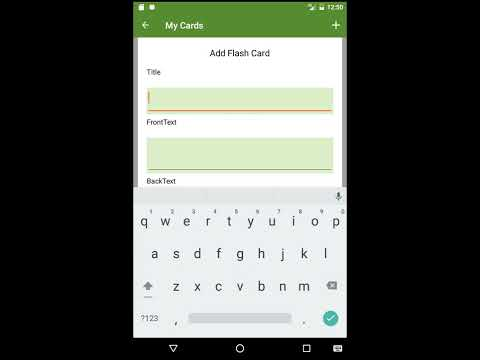# MATHXL:Vedic Maths, Mental math tricks & FlashcardEveryone
60
Offers in-app purchasesVedic Math MATHXL is a free android math app available for phones and tablets. MathXL app would help you explore, learn and practice various math tricks with video tutorials, flashcards and practice questions. MATHXL vedic maths covers all the topics required for competitive exams. MATHXL provide quick tricks which will improve your speed and precision required for exams.

Why MATHXL Vedic Math:
- Cover tricks for fast calculation over conventional methods
- Collection of ~200+ video tutorials for vedic math and mental math

MATHXL vedic math covers following topics:
2. Vedic Math Subtraction
3. Vedic Math Special Multiplication methods
4. Vedic Math Squaring, Square Roots, Cube and Cube Roots
5. Vedic Math Division
7. Vedic Math Logarithms
8. Vedic Math Averages, Mixtures and Alligations - Ratios
9. Vedic Math Co-ordinate Geometry
10. Vedic Math Time, Speed, Distance, Work
11. Vedic Math Permutations and Combinations
12. Vedic Math Number Systems
13. Vedic Math Arithmetic
14. Vedic Math Geometry
15. Vedic Math Algebra

- Learn Math Tricks provide video based training for 15+ topics.

- More than 80+ free Flashcards to help remember math formulas
- More than 15+ topics explained as per competitive exams syllabus
- Unlimited free chapter, practice and aptitude test
- Real time exams simulation
- Provide past performances in graphical format
- Scratch pad for rough calculations

MATH XL App structure:

math app has following sections:
1. Chapter Quiz
2. Flashcards
3. Practice Test
4. Time based Practice Test
5. Aptitude Test

- Learn Math Tricks provide video based training for 15+ topics.
- Chapter Quiz provide chapter quiz for key Vedic Maths topics
- User can import flashcards and play flashcards
- User can create own flashcards
- Practice Test, Time based practice test and Aptitude Test provide real time exams simulator. This will help you to practice Vedic Maths tricks.

App sync questions and flashcards from large questions bank hosted on cloud.

Icons provided by https://icons8.com/

Mental math, Math Formulas
Collapse

Review Policy
4.2
60 total
5
4
3
2
1

## What's New

Bug Fixed
Collapse

Updated
October 17, 2018
Size
32M
Installs
5,000+
Current Version
1.0.2
Requires Android
5.0 and up
Content Rating
Everyone
In-app Products
\$0.99 per item
Permissions
Offered By
Wiztech Digital Inc. Travel & Education
Developer